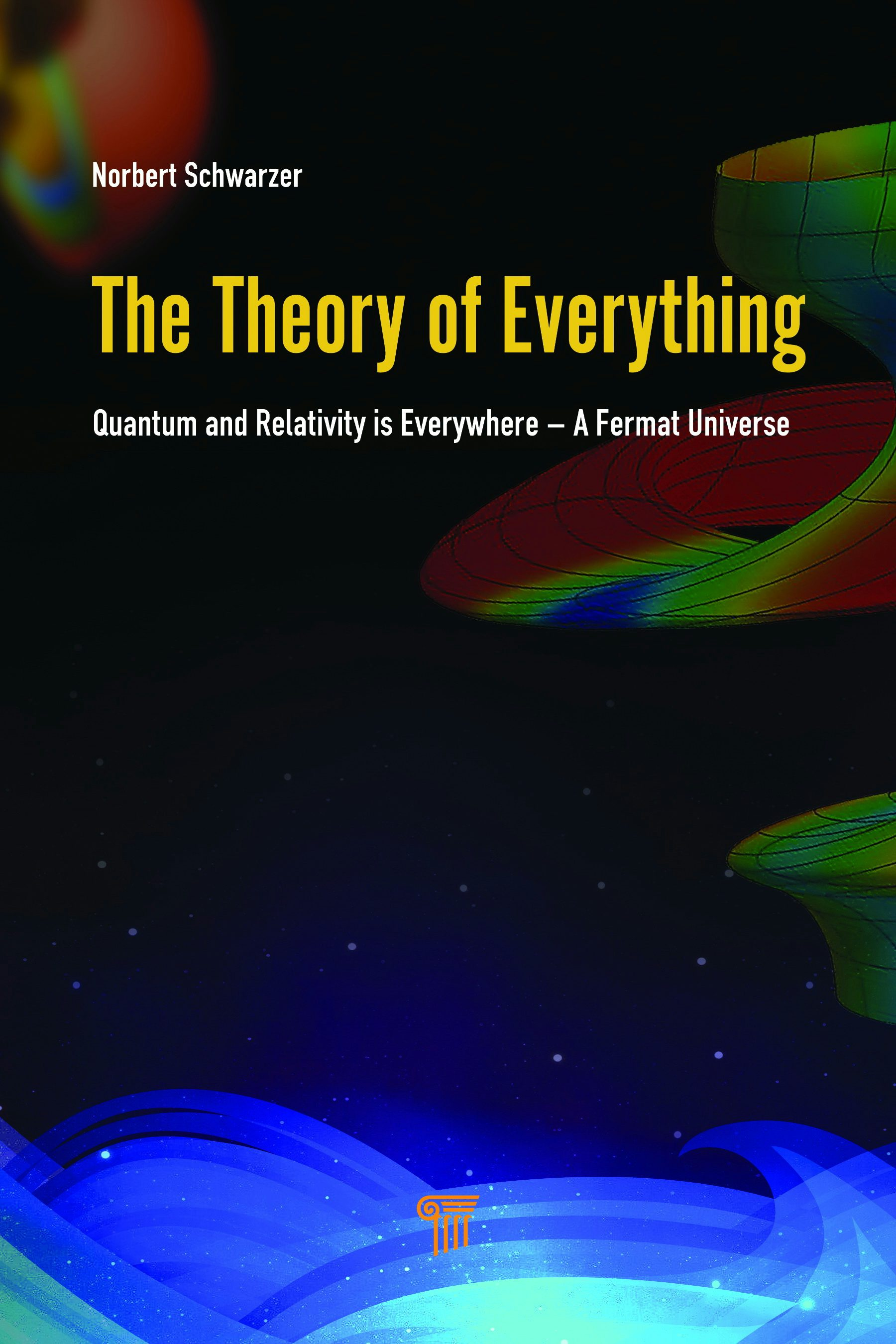# The Theory of Everything

## Quantum and Relativity is Everywhere – A Fermat Universe

Quoting  Richard P. Feynman—‘I think we can safely assume that no one understands quantum mechanics’—I am convinced that he would have made the following comments: (1) The Theory of Everything by Norbert Schwarzer is a quantum leap forward regarding the understanding of quantum mechanics. (2) There is no more need for the interpretation of the Copenhagen Convention, nor for The Cellular Automaton Interpretation of Quantum Mechanics by Nobel Laureate Gerard ’t Hooft. (3) The derivation of the laws of quantum mechanics as a result of the Einstein field equations is absolutely ingenious.

Prof. Hans Leuenberger, University of Basel, Switzerland
• Format: eBook
• ISBN: 9781315099750
• Subject: Mathematical Physics
• Published: March 2020

This book unifies quantum theory and the general theory of relativity. As an unsolved problem for about 100 years and influencing so many fields, it is important to the scientific community. Examples like Higgs field, limit to classical Dirac and Klein–Gordon or Schrödinger cases, quantized Schwarzschild, Kerr, Kerr–Newman objects, and the photon are considered for illustration. The book also provides an interesting explanation for the asymmetry of matter and antimatter in the early universe that the author found while quantizing the Schwarzschild metric.

Along the way, the methods outlined in the book are also used to tackle the problem of the proof of Fermat’s last theorem, because there is a connection between quantum theory and the basic mathematical laws of integers. The author shows that the proof of this theorem can be brought down to a few lines by applying new quantum theoretical methods. Because such proof has been sought for over 370 years, this book is of definite interest to mathematicians.